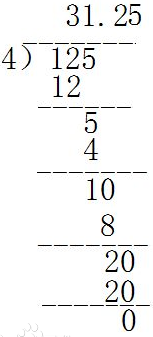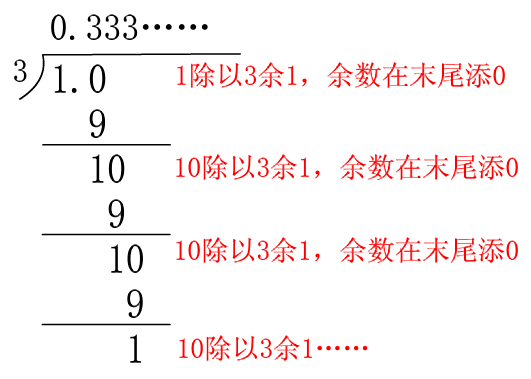## [USACO2.4]Fractions to Decimals【分数化小数】

PS: 一道经典的模拟题（或许以后高精会用上），需要用到数学的基本知识（如何判断循环节）

## 题目分析

注意：N/D是分数，分数属于有理数，有理数分为整数、有限小数和无限循环小数

1. $N$ 可以被 $D$ 整除（即 $N \div D$ 的值是一个整数），但输出需在末尾加上 $.0$ ，例如 $2 \div 2 = 1.0$ 。
2. $N$ 可以被 $D$ 除尽（即 $N \div D$ 的值是一个有限小数），例如 $55 \div 100 = 0.55$ 。
3. $N$ 不可以被 $D$ 除尽（即 $N \div D$ 的值是一个无限循环小数），循环节用一对小括号 $()$ 括住，例如 $1 \div 3=0.(3)$ （所以想用double的同学请绕道吧，无限小数是会爆精的）。

## 算法解析

• 首先，答案的整数部分可以直接通过 $N/D$ 算出，所以我们可以直接把字符串赋值为 $N/D$ 的值，然后再加一个小数点$(.)$，因为答案无论如何都有小数部分。

• 然后，就是最重要的除法模拟部分了。本题模拟的核心思路正是除法笔算（长这样的）由图可知，算商的小数部分时，会将上一次除下来的余数后面加上被除数的对应位的数字（如果没有就视作0）作为被除数，继续做除法。

现在，模拟思想问题已经解决了，但如何判断循环节又成了一道难关。因为小数部分的商与前面相同时也不一定是循环节， $555 \div 1000 = 0.555$ 就是一个经典的例子，它其实是一个有限小数，所以排除了用商作为判断条件的可能性。

有没有其他东西可以作为循环节的判断条件呢？答案是有的。通过观察我们发现，即使除出来的的商相同，商不是循环节的对应被除数（之前的余数）前面是找不到的，也就是循环节的数具有被除数相同的必然性。例如 $1 \div 3=0.3333……$ ，小数部分是这样的出来的：如图，循环节 $3$ 的被除数一直是 $10$ ，也就是之前算的余数一直是 $1$ ，余数相同。所以我们只要标记出现过的余数，如果有余数与之前的相同，那么之前余数作为被除数算出的商的位置直到算出这个余数的商的位置就是这个小数的循环节

解决了循环节的判断问题后，剩下的就简单了。

• 最后，就是输出了。输出也没什么难的，但要注意 $76$ 个字符换一行，不要忘记记上整数部分、小数点和小括号。